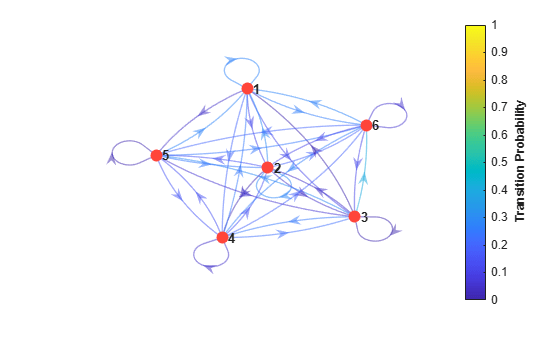# mcmix

Create random Markov chain with specified mixing structure

## Syntax

``mc = mcmix(numStates)``
``mc = mcmix(numStates,Name=Value)``

## Description

example

``mc = mcmix(numStates)` returns the discrete-time Markov chain `mc` containing `numStates` states. `mc` is characterized by random transition probabilities.`

example

````mc = mcmix(numStates,Name=Value)` uses additional options specified by one or more name-value arguments to structure `mc` to simulate different mixing times. For example, you can control the pattern of feasible transitions.```

## Examples

collapse all

Generate a six-state Markov chain from a random transition matrix.

```rng(1); % For reproducibility mc = mcmix(6);```

`mc` is a `dtmc` object.

Display the transition matrix.

`mc.P`
```ans = 6×6 0.2732 0.1116 0.1145 0.1957 0.0407 0.2642 0.3050 0.2885 0.0475 0.0195 0.1513 0.1882 0.0078 0.0439 0.0082 0.2439 0.2950 0.4013 0.2480 0.1481 0.2245 0.0485 0.1369 0.1939 0.2708 0.2488 0.0580 0.1614 0.0137 0.2474 0.2791 0.1095 0.0991 0.2611 0.1999 0.0513 ```

Plot a digraph of the Markov chain. Specify coloring the edges according to the probability of transition.

```figure; graphplot(mc,ColorEdges=true);```Generate random transition matrices containing a specified number of zeros in random locations. A zero in location (i, j) indicates that state i does not transition to state j.

Generate two 10-state Markov chains from random transition matrices. Specify the random placement of 10 zeros within one chain and 30 zeros within the other chain.

```rng(1); % For reproducibility numStates = 10; mc1 = mcmix(numStates,Zeros=10); mc2 = mcmix(numStates,Zeros=30);```

`mc1` and `mc2` are `dtmc` objects.

Estimate the mixing times for each Markov chain.

`[~,tMix1] = asymptotics(mc1)`
```tMix1 = 0.7567 ```
`[~,tMix2] = asymptotics(mc2)`
```tMix2 = 0.8137 ```

`mc1`, the Markov chain with higher connectivity, mixes more quickly than `mc2`.

Generate a Markov chain characterized by a partially random transition matrix. Also, decrease the number of feasible transitions.

Generate a 4-by-4 matrix of missing (`NaN`) values, which represents the transition matrix.

`P = NaN(4);`

Specify that state 1 transitions to state 2 with probability 0.5, and that state 2 transitions to state 1 with the same probability.

```P(1,2) = 0.5; P(2,1) = 0.5;```

Create a Markov chain characterized by the partially known transition matrix. For the remaining unknown transition probabilities, specify that five transitions are infeasible for 5 random transitions. An infeasible transition is a transition whose probability of occurring is zero.

```rng(1); % For reproducibility mc = mcmix(4,Fix=P,Zeros=5);```

`mc` is a `dtmc` object. With the exception of the fixed elements (1,2) and (2,1) of the transition matrix, `mcmix` places five zeros in random locations and generates random probabilities for the remaining nine locations. The probabilities in a particular row sum to 1.

Display the transition matrix and plot a digraph of the Markov chain. In the plot, indicate transition probabilities by specifying edge colors.

`P = mc.P`
```P = 4×4 0 0.5000 0.1713 0.3287 0.5000 0 0.1829 0.3171 0.1632 0 0.8368 0 0 0.5672 0.1676 0.2652 ```
```figure; graphplot(mc,'ColorEdges',true);```## Input Arguments

collapse all

Number of states, specified as a positive integer.

If you do not specify any name-value arguments, `mcmix` constructs a Markov chain with random transition probabilities.

Data Types: `double`

### Name-Value Arguments

Specify optional pairs of arguments as `Name1=Value1,...,NameN=ValueN`, where `Name` is the argument name and `Value` is the corresponding value. Name-value arguments must appear after other arguments, but the order of the pairs does not matter.

Before R2021a, use commas to separate each name and value, and enclose `Name` in quotes.

Example: `Zeros=10` places `0` at 10 random locations in the transition matrix.

Locations and values of fixed transition probabilities, specified as a `numStates`-by-`numStates` numeric matrix.

Probabilities in any row must have a sum less than or equal to `1`. Rows that sum to `1` also fix `0` values in the rest of the row.

`mcmix` assigns random probabilities to locations containing `NaN` values.

Example: ```Fix=[0.5 NaN NaN; NaN 0.5 NaN; NaN NaN 0.5]```

Data Types: `double`

Number of zero-valued transition probabilities to assign to random locations in the transition matrix, specified as a positive integer less than `NumStates`. The `mcmix` function assigns `Zeros` zeros to the locations containing a `NaN` in `Fix`.

Example: `Zeros=10`

Data Types: `double`

Unique state labels, specified as a string vector, cell vector of character vectors, or numeric vector of `numStates` length. Elements correspond to rows and columns of the transition matrix.

Example: ```StateNames=["Depression" "Recession" "Stagnant" "Boom"]```

Data Types: `double` | `string` | `cell`

## Output Arguments

collapse all

Discrete-time Markov chain, returned as a `dtmc` object.

 Gallager, R.G. Stochastic Processes: Theory for Applications. Cambridge, UK: Cambridge University Press, 2013.

 Horn, R., and C. R. Johnson. Matrix Analysis. Cambridge, UK: Cambridge University Press, 1985.